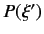# Measurements

We have seen that a measurement of some observable $$\xi$$ of a microscopic system causes the system to jump into one of the eigenstates of $$\xi$$. The result of the measurement is the associated eigenvalue (or some function of this quantity). It is impossible to determine into which eigenstate a given system will jump, but it is possible to predict the probability of such a transition. So, what is the probability that a system in some initial state $$\mid B \rangle$$ makes a transition to an eigenstate $$\mid \xi' \rangle$$ of an observable $$\xi$$, as a result of a measurement made on the system? Let us start with the simplest case. If the system is initially in an eigenstate $$\mid \xi' \rangle$$ then the transition probability to a eigenstate $$\mid \xi'' \rangle$$ corresponding to a different eigenvalue is zero, and the transition probability to the same eigenstate $$\mid \xi' \rangle$$ is unity. It is convenient to normalize our eigenkets such that they all have unit norms. It follows from the orthogonality property of the eigenkets that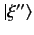(50)

where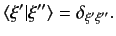is unity if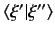, and zero otherwise. For the moment, we are assuming that the eigenvalues of $$\xi$$ are all different.

Note that the probability of a transition from an initial eigenstate $$\mid \xi' \rangle$$ to a final eigenstate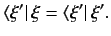is the same as the value of the inner product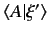. Can we use this correspondence to obtain a general rule for calculating transition probabilities? Well, suppose that the system is initially in a state $$\mid B \rangle$$ which is not an eigenstate of $$\xi$$. Can we identify the transition probability to a final eigenstate $$\mid \xi' \rangle$$ with the inner product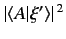? The straight answer is no'', sinceis, in general, a complex number, and complex probabilities do not make much sense. Let us try again. How about if we identify the transition probability with the modulus squared of the inner product,? This quantity is definitely a positive number (so it could be a probability). This guess also gives the right answer for the transition probabilities between eigenstates. In fact, it is the correct guess.

Since the eigenstates of an observable $$\xi$$ form a complete set, we can express any given state $$\mid B \rangle$$ as a linear combination of them. It is easily demonstrated that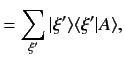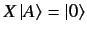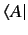(51)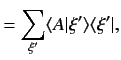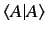(52)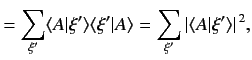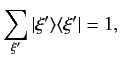(53)

where the summation is over all the different eigenvalues of $$\xi$$, and use has been made of Eq. (20), and the fact that the eigenstates are mutually orthogonal. Note that all of the above results follow from the extremely useful (and easily proved) result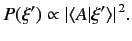(54)

where 1 denotes the identity operator. The relative probability of a transition to an eigenstate $$\mid \xi' \rangle$$, which is equivalent to the relative probability of a measurement of $$\xi$$ yielding the result $$\xi'$$, is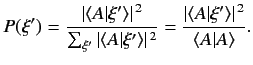(55)

The absolute probability is clearly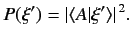(56)

If the ket $$\mid B \rangle$$ is normalized such that its norm is unity, then this probability simply reduces to